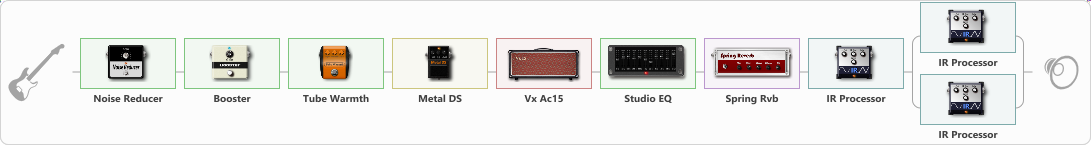Discussion in 'ToneLib-GFX presets' started by Tony the Metalhead, Mar 21, 2022.

1. ### Tony the MetalheadActive Member

Let me know if you enjoy this toneEffects chain:Effect: "Noise Reducer" (Dynamics / Filter), active - "yes"
{
"Sens" = 40
"Mode" = Soft
}

Effect: "Booster" (Dynamics / Filter), active - "yes"
{
"Gain" = 25
}

Effect: "Tube Warmth" (Dynamics / Filter), active - "yes"
{
"Input" = 100
"Warmth" = 65
"Level" = 100
}

Effect: "Metal DS" (Overdrive / Distortion), active - "yes"
{
"Dist" = 20
"Bass" = 50
"Middle" = 30
"Treble" = 70
"Level" = 100
}

Effect: "Vx Ac15" (Amp simulators), active - "yes"
{
"Gain" = 60
"Bass" = 15
"Middle" = 10
"Treble" = 60
"Presence" = 35
"Master" = 100
"Level (dB)" = 0
}

Effect: "Studio EQ" (Dynamics / Filter), active - "yes"
{
"31 Hz" = -4
"62 Hz" = -5
"125 Hz" = -4
"250 Hz" = -5
"500 Hz" = -5
"1 kHz" = -4
"2 kHz" = 6
"4 kHz" = 10
"8 kHz" = 8
"16 kHz" = 0
"Level (dB)" = 0
}

Effect: "Spring Rvb" (Reverberation), active - "yes"
{
"Time" = 4.0
"PreDelay" = 0
"LoDamp" = 0
"HiDamp" = 100
"Mix" = 40
}

Effect: "IR Processor" (Cabinets), active - "yes"
{
"IR" = king_nothing_IR
"Low Cut (Hz)" = 80
"Hi Cut (kHz)" = 20.0
"Mix" = 100
"Level (dB)" = 0
}

Effect: "Splitter" (Dynamics / Filter)
{
"A-Bypass" = Off
"A-Pan" = -25
"A-Level" = 55
"B-Bypass" = Off
"B-Pan" = 25
"B-Level" = 55
"Width" = 0

'A' branch:
{

Effect: "IR Processor" (Cabinets), active - "yes"
{
"IR" = OD-R112-V30-SM58-P05-20
"Low Cut (Hz)" = 80
"Hi Cut (kHz)" = 8.0
"Mix" = 100
"Level (dB)" = 0
}
}
'B' branch:
{

Effect: "IR Processor" (Cabinets), active - "yes"
{
"IR" = OD-R112-V30-SM58-P09-40
"Low Cut (Hz)" = 120
"Hi Cut (kHz)" = 20.0
"Mix" = 100
"Level (dB)" = 0
}
}
}

Note: You will need to download and install the ToneLib-GFX software to use the preset.